Udacity’s ‘Introduction to Physics’ course

# Udacity’s ‘Introduction to Physics’ course

Took ‘Introduction to Physics’ course at Udacity. It is a beginner level course and I have skimmed through most of it. But what kept me going till the end is the extremely engaging way in which it is taught. Every lesson, you take one important Physics problem and try to solve it. You make progress in small steps by learning required techniques and mathematics. Interactive nature of the platform always makes you think by asking questions before introducing new concepts. On top that, all this is linked with the history of the problem, who solved it and how?

The course has 7 lectures:

### Lecture # 1: Circumference of Earth

The Greek philosopher, Eratosthenes (276 BC – 195 BC), was the first person to calculate the circumference of earth with remarkable accuracy. He used geometry and trigonometry to figure this out.

He only needed to take following measurements for his calculation:

• Length of the shadow cast by an object A at noon at location X.
• Height of the object A.
• Distance of location X to the nearest location where sun doesn’t cast any shadow at noon.

See the diagram and link below for details.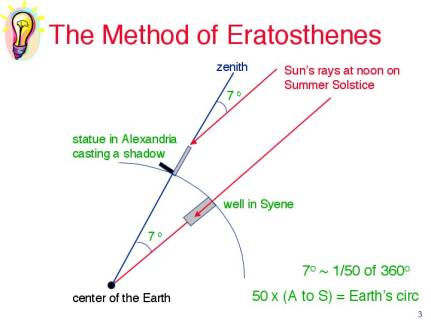### Lecture # 2: Speed of freely falling object

Aristotle had following view on this:

• Objects fall at constant speed.
• Speed is directly proportional to mass of object.

It was Galileo who found that the distance covered by a falling object is directly proportional to the square of time it has been falling (i.e. objects fall with constant acceleration irrespective of their mass).

### Lecture # 3: What causes motion?

The prevalent view before Galileo and Newton was Aristotelian. According to which there is one set of laws governing heavenly bodies and a different set for bodies on earth.

Newton through his laws of motion asserted that both heavens and the earth follow the same laws. And the apple falling from a tree or moon orbiting the earth do so under the influence of same force.

### Lecture # 4: Keeping Holland DryThis lecture is about Archimedes Screw and the basic principles behind its working. It builds on the understanding of force from previous lectures and introduces simple machines: Inclined Plane, Pulley and Lever. Simple machines enable us to trade off the force required to lift or move things with the distance. For example, using an inclined plane to move something to a higher level will require less force but we need to push it for a longer distance.

Next, the concepts of Work, Energy and Power are introduced.

• Work = Force * Distance
• Work = Change in Energy
• Power = Work / Time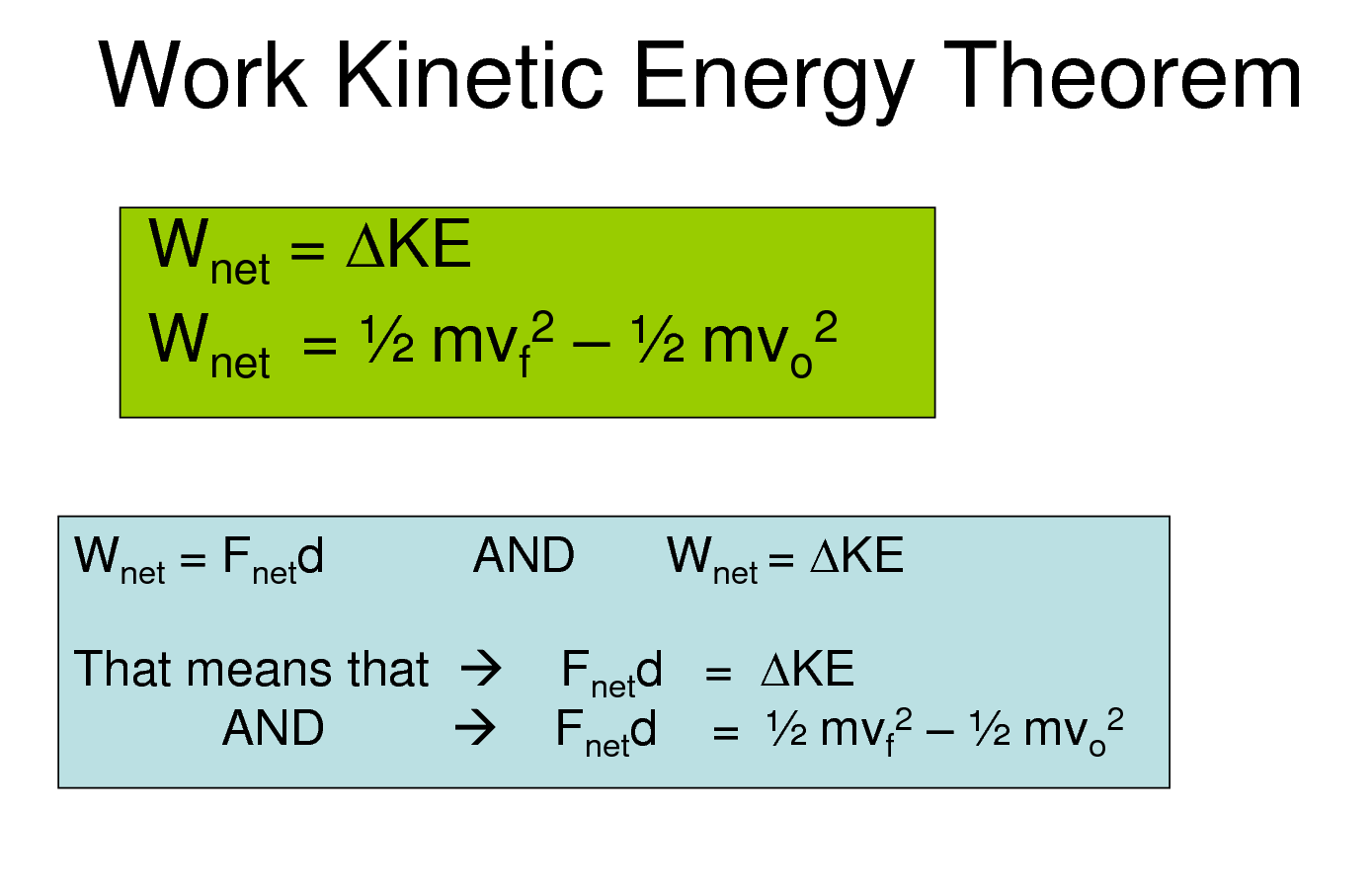Finally, the Law of Conservation of Energy is discussed with the example of roller coaster ride. During the ride, energy changes its form between kinetic and potential with some of the energy lost due to friction as heat.

### Lecture # 5: Lost at Sea

Problem of this lecture is how to measure latitude and longitude?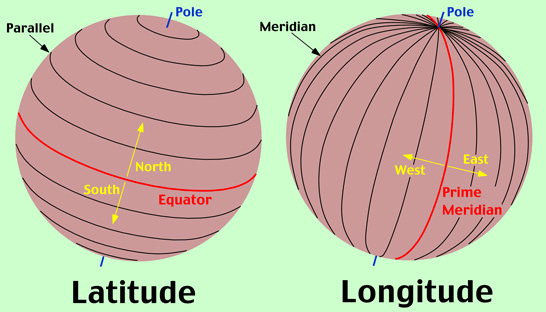Latitude is relatively easier to calculate while longitude is much trickier.

When sun is at the highest point in the sky and it is equinox, the latitude can be calculated as explained in the first lecture.

For calculating Longitude, we need to keep track of time. Galileo attempted to measure time by observing transit of Jupiter’s moon but the idea was not very fruitful due to the difficulties involved.

The answer lies in Simple Harmonic Motion.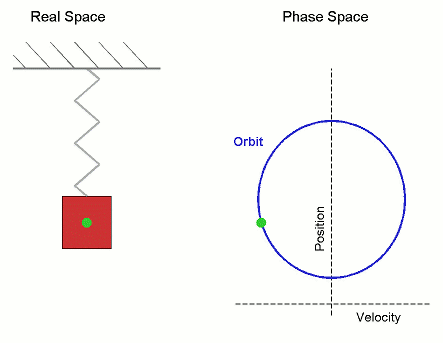Elastic Potential Energy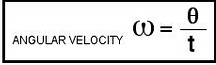k = Spring Constant

Lecture discusses the discovery of Pendulum by Galileo and invention of Pendulum Clock by Christiaan Huygens.

Longitude can be measured by comparing the time on ship to the time of a known location. Pendulum clocks can be used to reliably measure time and hence calculate longitude.

### Lecture # 6: Lightning Strikes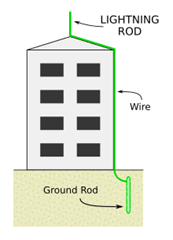Lightning is an electrical phenomenon. This was not very clear in 18th century. The link between lightning and electricity was there in the literature but it was Benjamin Franklin (17-Jan-1706  to  17-Apr-1790) who emphasized this link and developed lightning rods which prevented buildings from burning due to lightning strikes.

This lecture talks about basics of electricity with particular focus on lightening rod and how it grounds the electric current keeping buildings safe.

### Lecture # 7: Special Relativity

This is brief teaser to Special Relativity explaining the following two postulates of the theory and how they lead to bizarre conclusions like time dilation.

• Laws of Physics are same in all inertial frame of reference
• Speed of light constant regardless of the frame of reference

de Sitter double star experiment is explained as test of Special Relativity.

It concludes with a final exam where you can test your problem solving skills using the knowledge gained through the course. There are couple of bonus lectures also about ‘simple harmonic motion’ and ‘why can’t we open windows on an airplane’.